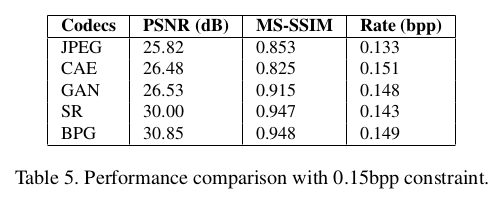# 论文引入

• 基于CAE，GAN，SR提出了三种整体压缩体系结构
• 对这三种框架做了全面的性能比较

# CAE用于图像压缩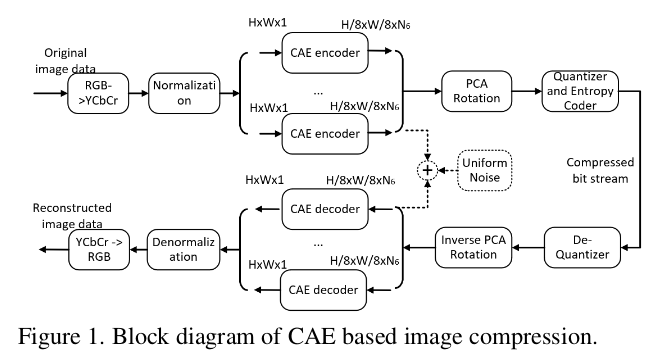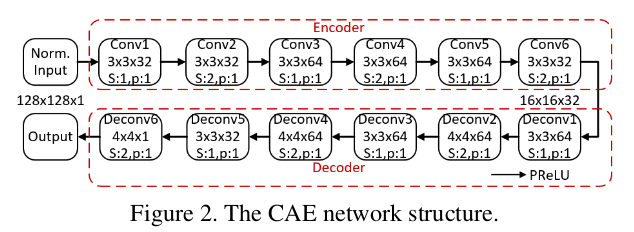$\begin{eqnarray} J\left(\theta,\phi;x\right) &=& \Vert x - \hat{x} \Vert^2 + \lambda \cdot \Vert y \Vert^2\\ &=& \Vert x - g_{\phi}(f_{\theta}(x)+\mu)\Vert^2 + \lambda \cdot \Vert f_{\theta}(x) \Vert^2 \end{eqnarray}$

# GAN用于图像压缩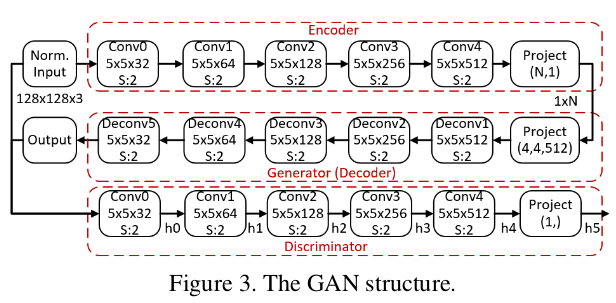$J_G(x) = \Vert x - \hat{x} \Vert^2 + \beta \sum_{i \in [0,4]}\Vert D_{hi}(x) - D_{hi}(\hat{x}) \Vert^2$

# SR用于图像压缩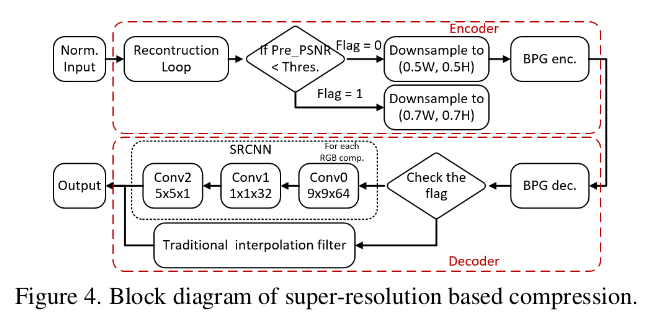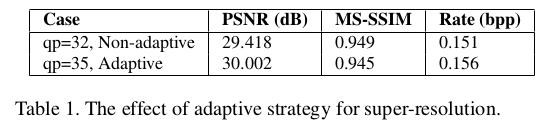# 性能比较

CAE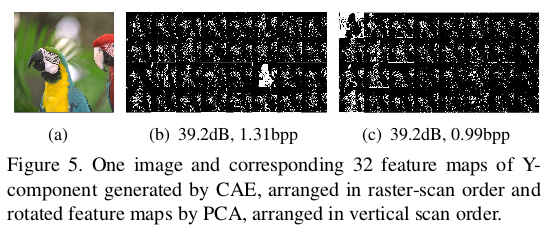GAN

GAN的图像压缩在CLIC验证数据集上进行了性能比较实验：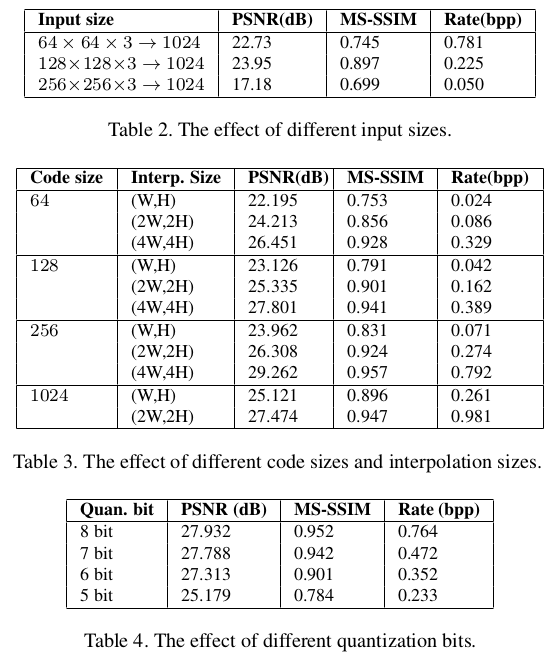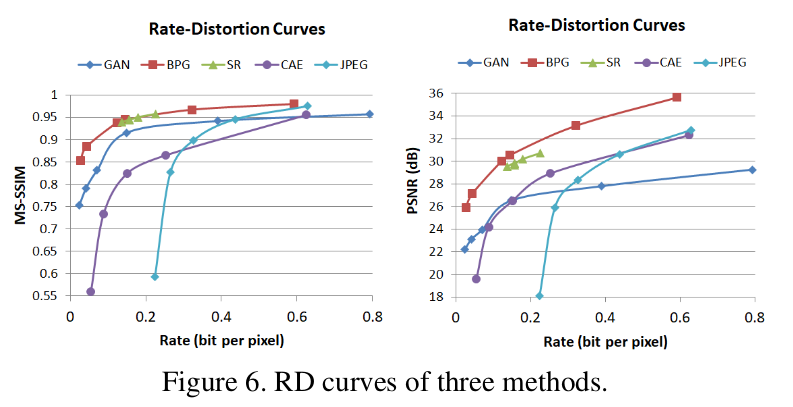• 由于自动编码器的固有特性，在有损压缩的情况下，CAE优于JPEG。自动编码器可以减少尺寸以从图像中提取压缩的演示文稿，因此CAE优于JPEG和JPEG2000。
• GAN在低比特率下比在高比特率下表现更好，因此GAN倾向于实现大的压缩比。同时，GAN在MS-SSIM上的性能优于PSNR，因为GAN的重建是基于图像数据的分布，肉眼更加认同。特别是对于MS-SSIM，GAN具有从0.2bpp到0.8bpp的稳定性能。
• SR在这三种方法中实现了最佳性能，因为它具有新兴算法BPG和基于机器学习的超分辨率滤波器的优点。如果可以提供更多的计算资源，那么通过添加更好的超分辨率滤波器，可以预期有希望的结果将超过BPG。